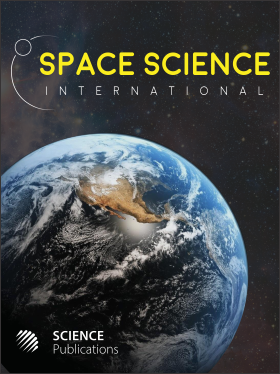Frequency: Continuous
ISSN: 2524-2253 (Print)
ISSN: 2524-2261 (Online)
Research Article Open Access

# Non-Singular Thermodynamic Gravity

Pharis E. Williams1
• 1 New Mexico Tech., United States

## Abstract

Newton formed his laws of motion, but still had to assume a law of gravity in order to determine the motions of bodies interacting through gravitational forces. Newton gave a prescription of how to find the dynamics produced by gravity. Einstein sought to determine the motions of gravity without using a force. Rather, he sought a system of equations by which the dynamics of bodies interacting through gravity were determined by the least distance between two points in a curved space. In both of these approaches gravity was basically independent of other forces of nature. Newton’s approach was to provide a system of equations that determined the motion of bodies interacting through forces in any choice of geometry. Einstein’s approach was to require that the force of gravity determine the geometry since he sought to find the motions without using a force. Newton gave three laws of motion in equations that could be used to find various types of energy such as potential energy or kinetic energy. This arrangement has the forces determining the energy of interactions. The approach presented here basically reverses these roles of energy and forces. It starts with a law stating the conservation of energy which states how any exchange of energy between a system and its surroundings affects the system’s energy and the work it can perform. It is a law stating how these three energies, exchange energy, system energy and work, can interact. The expression for work, force acting through a distance, must be a path dependent term which also makes the exchange energy path dependent. However, the system’s energy is not path dependent. The difference between the path dependence of the work and the path independence of the system’s energy is shown to be crucial to the law’s determination of dynamics.

Space Science International
Volume 1 No. 2, 2013, 63-76

DOI:

Submitted On: 19 October 2013 Published On: 23 December 2013

How to Cite: Williams, P. E. (2013). Non-Singular Thermodynamic Gravity. Space Science International, 1(2), 63-76. https://doi.org/10.3844/ajssp.2013.63.76

• 1,935 Views